3. # Implementing Equals

Implementing Logical Operators and Equality

We’ve now dealt with the arithmetic operators, so let’s continue by considering the logical and comparison operators. As you’ve already seen, C++ provides a set of comparison operators, and they’re summarized in the following table.

 Operator Description == Equality != Inequality > Greater than >= Greater than or equal to < Less than <= Less than or equal to Table 3

Implementing these operators is quite simple and follows the model of the addition operator in the previous exercises. Here’s how to implement the equality operator (==):

8.        Using the same project as in the previous exercises, find the operator+ function in your code and add the following function after it:

// The equality operator for the Dbl struct

static bool operator==(Dbl lhs, Dbl rhs)

{

return lhs.val == rhs.val;

}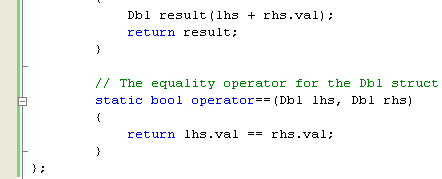The function follows the same pattern as those you implemented for the arithmetic operators. It is a static member of the Dbl type, but this time, it returns a Boolean, just as you’d expect a logical operator to do, and it makes its decision by comparing the inner structure of its two operands.

What Is Equality?

Deciding whether to implement == and != depends on the type you’re writing, and it might not be a simple decision. For some classes, the choice is fairly obvious: take a Point type, which has x and y members. In this case, == would compare the x and y members of two Points and return true if they’re the same. What about a Currency class that has a value and a currency type? You might say that two Currency objects are the same if both the value and the currency type are identical. Likewise, you might say that the two objects are identical if their values are the same when converted to some underlying base currency, such as dollars or Euros. Both view points are equally valid; it’s up to you to choose one and document it.

Additionally, there might be classes for which any notion of equality is artificial. Consider a BankAccount class: what would equality mean? Two account objects can’t be identical because they have different, unique account numbers. You might choose some criteria that you could test for equality, or you might decide that equality doesn’t apply to BankAccount objects. As a final point, you should be aware that testing for equality can pose problems for floating-point values. Some values can require more decimal places than the float and double types support, so there might be rounding errors due to rounding the final decimal place up or down. It’s quite possible that rounding errors could mean that two identical values will apparently be unequal. One way around this problem is to define a class, such as Dbl, that makes allowances for this situation by testing whether the difference between two values falls within a given tolerance. To provide a more realistic test, you can code the equality operator for Dbl like this:

// A better equality operator for the Dbl struct

static bool operator==(Dbl lhs, Dbl rhs)

{

if (Math::Abs(lhs.val - rhs.val) < 0.00001)

return true;

else

return false;

}

The Math::Abs method is a static member of the Math class that returns an absolute value.

9.        Add some test code to the main() function to test the new operator.

Console::WriteLine(); // blank line

if (d1 == d2)

Console::WriteLine("d1 and d2 are equal");

else

Console::WriteLine("d1 and d2 are not equal");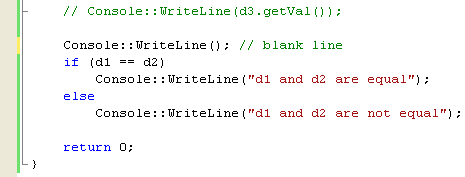10.     As you’d expect, d1 and d2 aren’t equal, so if you compile and run your program, you see the second message.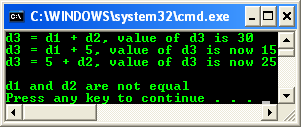You can take a shortcut when you implement the inequality operator (!=) by making use of the fact that its result is the opposite of whatever == would return for the same operands. So, you can implement the inequality operator in terms of the equality operator. Add the following code after the overload for operator==:

// The inequality operator for the Dbl struct

static bool operator!=(Dbl lhs, Dbl rhs)

{

return !(lhs == rhs);   // calls operator!=()

}The function compares its two arguments using the == operator, which causes the operator== function to be called. The operator!= function then applies the not operator (!) to turn a true result into false, and vice versa. In this case, using the shortcut doesn’t save us any code, but if operator== ended up checking a lot of data members, this shortcut could be worthwhile. It will also help if the structure of the class changes because you’ll only have to change the implementation of operator==.

The other logical operators (<, <=, >, and >=) can be overloaded in a similar manner, and you can make use of the same shortcuts when implementing pairs of operators.

Implementing Equals

You’ve already learned that all types in .NET ultimately inherit from the Object class. This class provides several functions that all .NET types inherit, and one in particular is relevant to our discussion of the == and != operators: Equals. The Object::Equals method is intended to let types provide a way of comparing content, as opposed to comparing references. This is the same task that you’ve performed by implementing the == operator. However, one of the great attractions of .NET is that managed types can be accessed seamlessly from other languages, and other languages might well want to use Equals to test for equality. The following exercise shows you how to implement Equals for the Dbl struct.

1.        Using the same project as in the previous exercises, find the operator!= function in your code and add the following function after it:

// The equality operator for the Dbl struct

// The Equals() function for the Dbl struct...

// override the base class (coz the original is Equals(x, y),

// here only 1 argument, then it need virtual keyword...

// Using implicit boxing

virtual bool Equals(Object^ pOther) override

{

// cast pOther to Dbl

Dbl^ s = dynamic_cast<Dbl^>(pOther);

// test whether it is the null pointer

if (s == nullptr)

return false;

// else...

else

return s->val == val;

}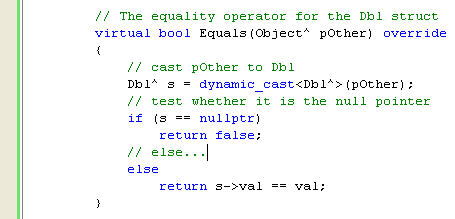This operator function is more complex than others in this module, so let’s look at it line by line. The first line declares the Equals function to take an Object^ as its single argument and return a bool. It’s important that you declare it exactly this way; otherwise, it won’t be an override for the virtual function inherited from the Object base class. The use of Object^ for the argument type means that you can pass a pointer to any managed type into the function, so the first thing you need to do is to make sure that it is actually a Dbl* that has been passed in. You run this test by using a dynamic cast, dynamic_cast. The dynamic cast is a mechanism introduced by C++ that allows you to perform a cast at run time. Here the cast is from Object^ to Dbl^. If the cast fails, if the pointer passed in isn’t a Dbl^, the result will be a null pointer (nullptr). The function checks for this possibility and returns false if it is the case. If the dynamic cast works, you know that you have a Dbl ^; therefore, you can check its content to see if it is the same as that of the object. Calling the Equals method from code is more complicated than using the operators we’ve already met because of the need to pass in an Object^ pointer.

2.        Add the following code to the main() function after your test of the == operator:

if (d1.Equals((Object^)d3))

Console::WriteLine("d1 and d3 are equal");

else

Console::WriteLine("d1 and d3 are not equal");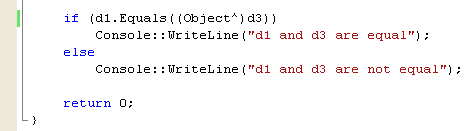3.        Build and run your project. The sample output is shown below.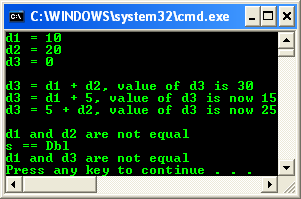This code calls the Equals function on d1 to see whether d1 is equal to d3. Equals needs a pointer to an Object, so you need to somehow get an Object^ representing d3 (implicit boxing). You do so by using the keyword Object^. You learned how value types are as efficient as built-in types, but they can also be used as reference types when the need arises. Treating a value type as a reference type is done by boxing it. Boxing is basically involves wrapping an object around a value type. The object wrapper is a reference type and acts as a box to contain the value. This process might seem complicated, but you’re really providing Equals for other .NET languages to use. In managed C++ code, you’d usually use == instead, without worrying about boxing. If you implement Equals for a type, it’s strongly recommended that you implement the == operator.